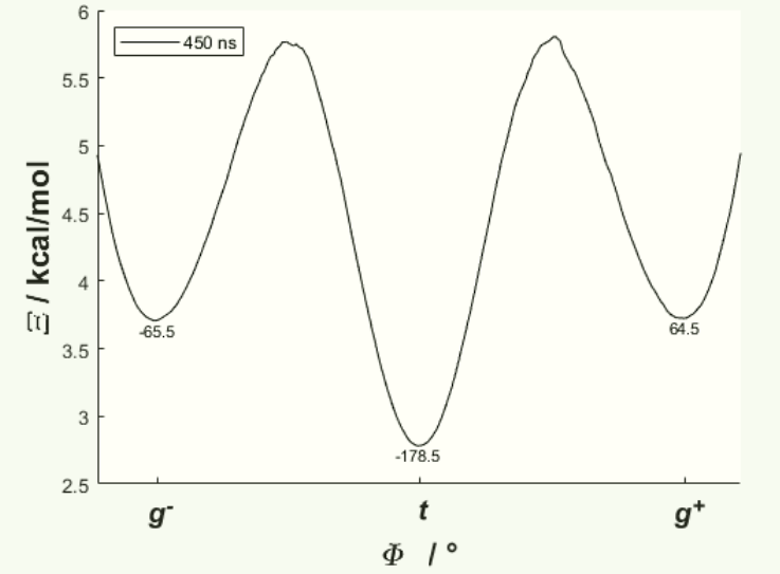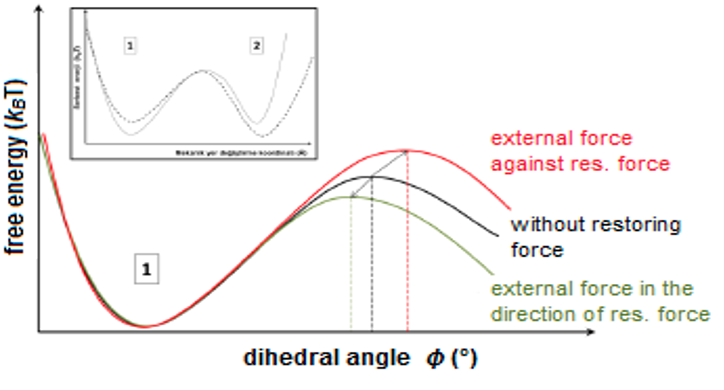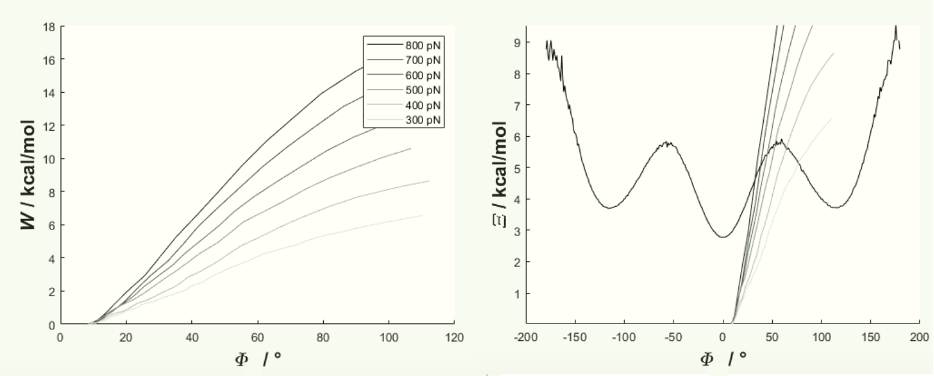# Unraveling Conformational Surfaces of Small Molecules

Term:
2021-2022 Fall
Faculty Department of Project Supervisor:
Faculty of Engineering and Natural Sciences
Number of Students:
3

The free energy (FE) surface (Figure 1) with respect to one or more collective variables is proportional to -RTln[P], where P is the equilibrium probability distribution (PD) for a chemical system with respect to that specific collective variable.  Alas, obtaining an ergodic probability distribution for systems of increasing atomic count becomes increasingly impractical, so there have been many methods developed for approximating FE.Figure 1.  FE Surface of Pentane versus its torsional angle.  t, g+, and g- denote the three thermodynamically stable conformations, whose values are written on the figure.  PD obtained from a 450 ns MD simulation.Figure 2. Illustration of Bell’s Theorem: the application of an external force shifts the onset and changes the height of a free energy barrier proportionately.

Bell’s Theorem (Bell 1978) states that energy barriers change height and shift upon the application of an external mechanical force (Figure 2).  In this project, you will run non-equilibrium Steered Molecular Dynamics (SMD) simulations for various conformational changes in various small molecules under varying magnitudes of force.  A sample output, the non-equilibrium work curves of varying magnitudes of force for the conformational change tt → tg+ of pentane, is shown in Figure 3.  Once we have amassed non-equilibrium work curves for several molecules, we will develop a general relationship/proportionality between magnitude of applied force and the real FE surface in Figure 1.Figure 3.  Left) Work done for a t  g+ conformational change of pentane in an SMD simulation.  Right) Those works superimposed onto the FE surface shown in Fig. 1 – the reference FE surface.

References:

Bell, G.  Models for the Specific Adhesion of Cells to Cells.  Science,1978, 200(4342), 618-627

University of Illinois at Urbana-Champaign. (2015, September) Stretching Deca-alanine. Retrieved August 12, 2020 from https://www.ks.uiuc.edu/Training/Tutorials/#namd

Related Areas of Project:
Molecular Biology, Genetics and Bioengineering
Materials Science ve Nano Engineering
Physics